Area of Square and Rectangles - Worksheet

Chapter 9 Class 7 Perimeter and Area
Serial order wise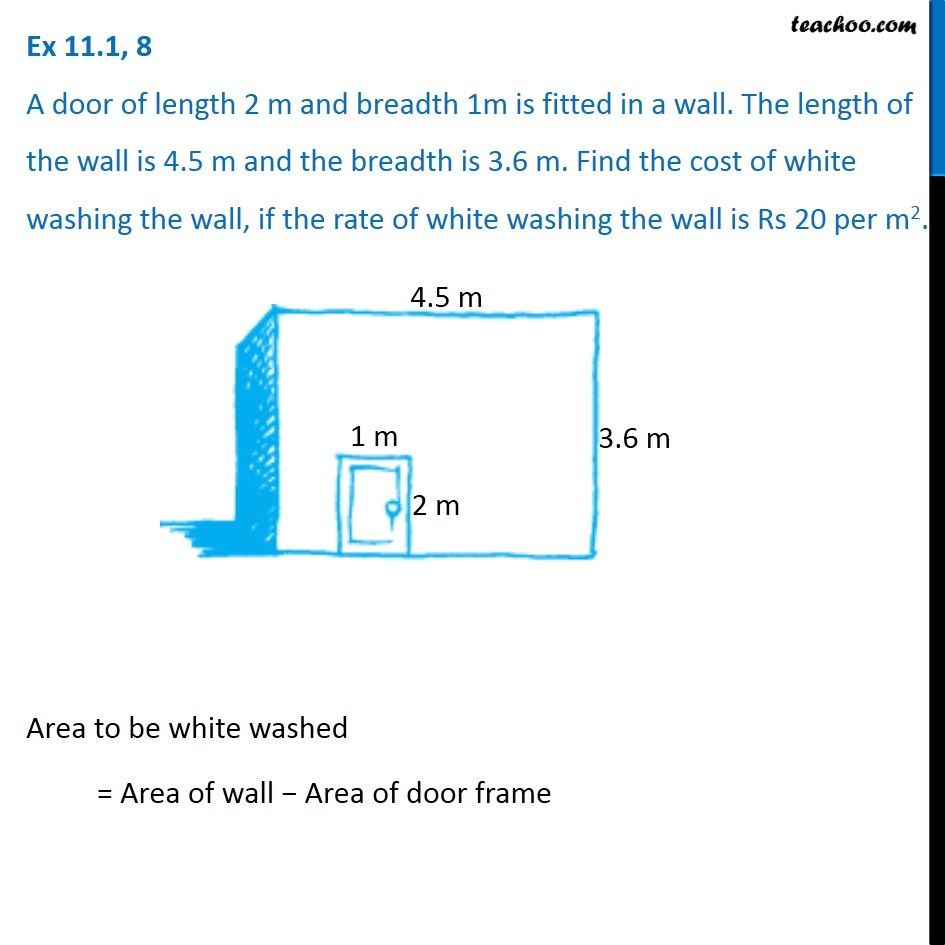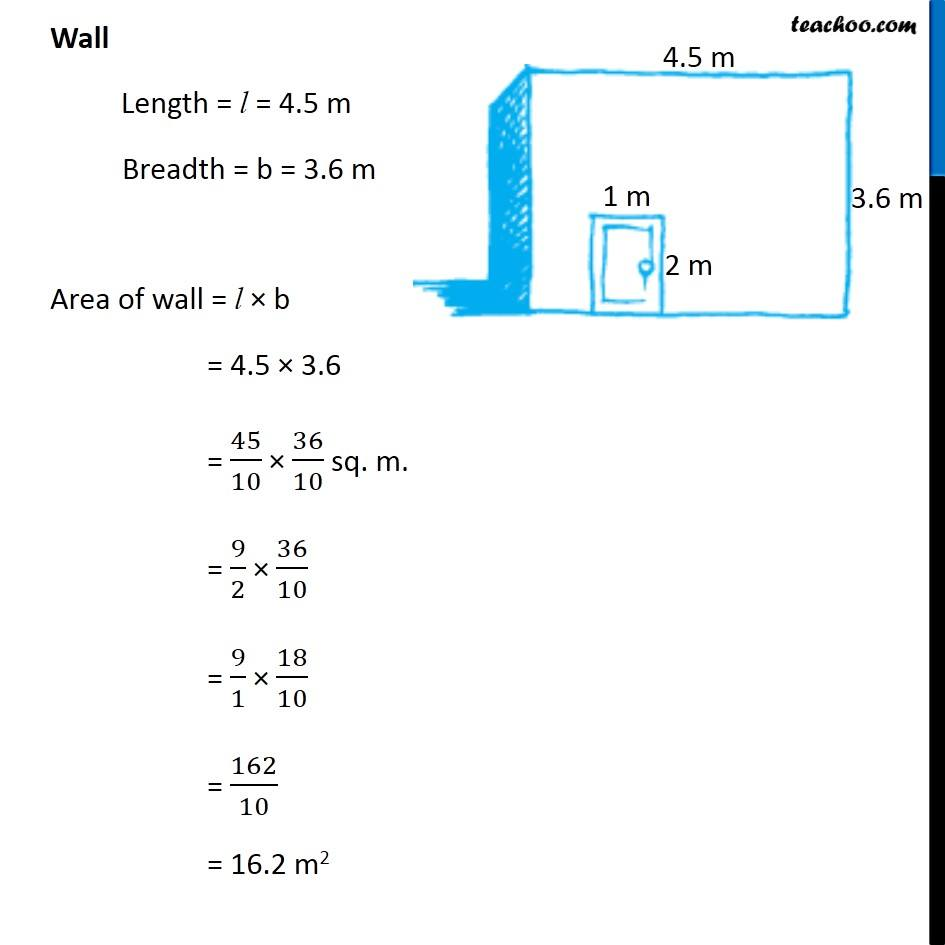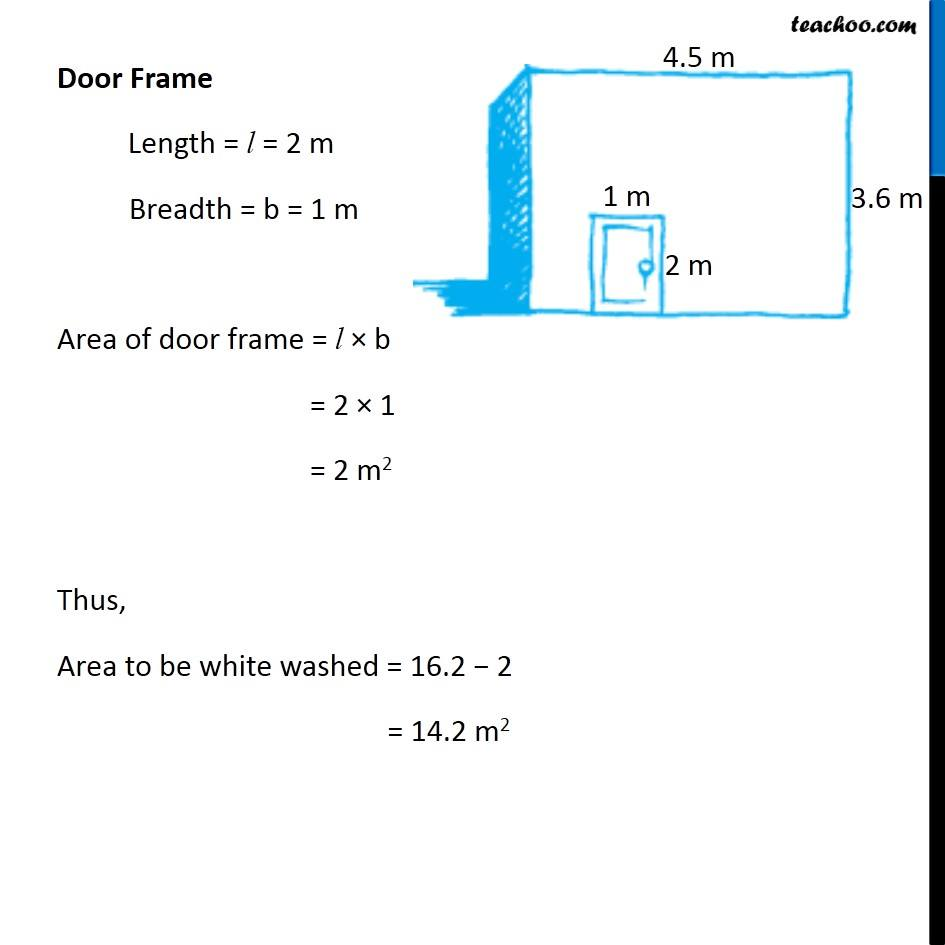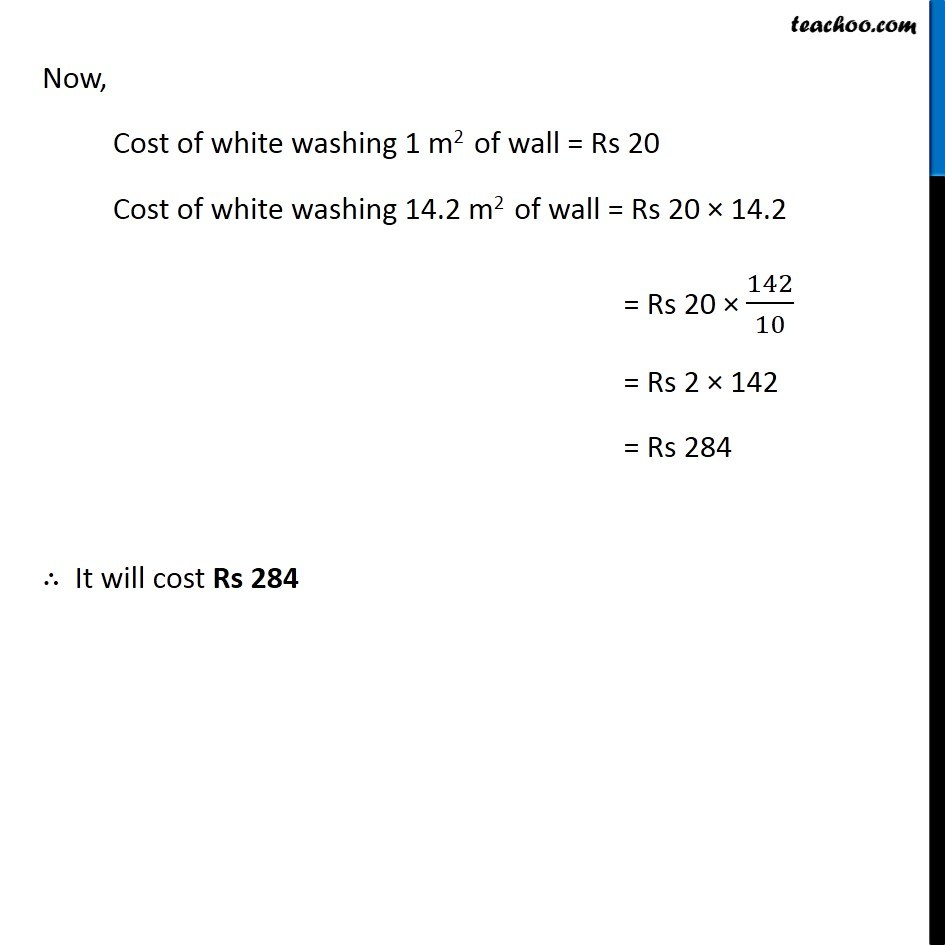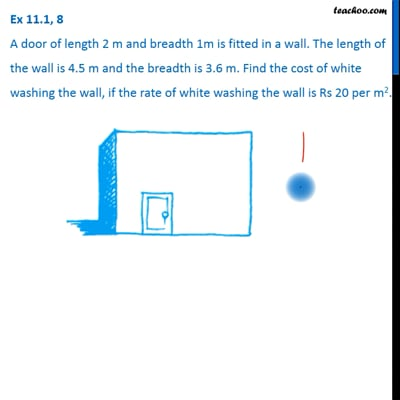This video is only available for Teachoo black users

Learn in your speed, with individual attention - Teachoo Maths 1-on-1 Class

### Transcript

Question 8 A door of length 2 m and breadth 1m is fitted in a wall. The length of the wall is 4.5 m and the breadth is 3.6 m. Find the cost of white washing the wall, if the rate of white washing the wall is Rs 20 per m2. Area to be white washed = Area of wall − Area of door frame Wall Length = l = 4.5 m Breadth = b = 3.6 m Area of wall = l × b = 4.5 × 3.6 = 45/10 × 36/10 sq. m. = 9/2 × 36/10 = 9/1 × 18/10 = 162/10 = 16.2 m2 Door Frame Length = l = 2 m Breadth = b = 1 m Area of door frame = l × b = 2 × 1 = 2 m2 Thus, Area to be white washed = 16.2 − 2 = 14.2 m2 Now, Cost of white washing 1 m2 of wall = Rs 20 Cost of white washing 14.2 m2 of wall = Rs 20 × 14.2 = Rs 20 × 142/10 = Rs 2 × 142 = Rs 284 ∴ It will cost Rs 284